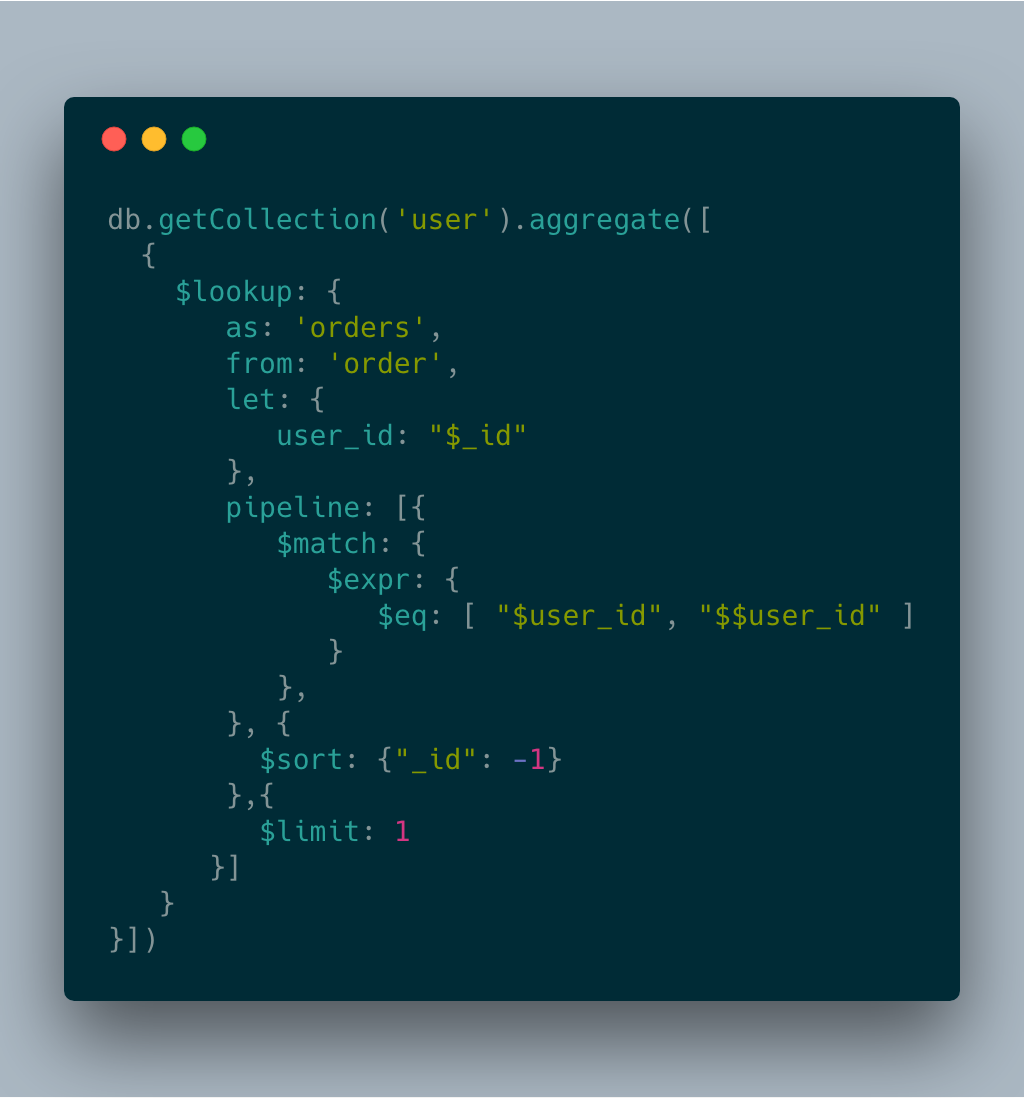# MongoDB 透過 lookup pipeline 實踐 Left Join``````user
| _id | name   |
|-----|--------|
| 1   | mlwmlw |
| 2   | wawa   |
| 3   | hap    |

order
| _id | user_id | amount |
|-----|---------|--------|
| 1   | 1       | 300    |
| 2   | 2       | 123    |
| 3   | 1       | 500    |``````

``````SELECT user._id, user.name, sum(order.amount) from user
LEFT JOIN order on user._id = order.user_id``````

``````db.getCollection('user').aggregate([
{\$lookup:
{
as: 'orders',
from: 'order',
localField: "_id",
foreignField: "user_id"
}
}])``````

``````//result
[
{
"_id" : 1,
"name" : "mlwmlw",
"orders" : [
{
"_id" : 1,
"user_id" : 1,
"amount" : "300"
},
{
"_id" : 3,
"user_id" : 1,
"amount" : 500
}
]
},
{
"_id" : 2.0,
"name" : "wawa",
"orders" : [
{
"_id" : 2,
"user_id" : 2,
"amount" : 123
}
]
},
{
"_id" : 3.0,
"name" : "hap",
"orders" : []
}
]``````

``Aggregate \$lookup Total size of documents in matching pipeline exceeds maximum document size``

``````db.getCollection('user').aggregate([
{\$lookup:
{
as: 'orders',
from: 'order',
let: {
//order.user_id
order_user_id: "\$user_id"
},
pipeline: [{
\$match: {
\$expr: {
// on user._id = order.user_id
\$eq: [ "\$_id", "\$\$order_user_id" ]
}
},
}, {
\$sort: {"_id": -1}
},{
\$limit: 1
}]

}
}])``````